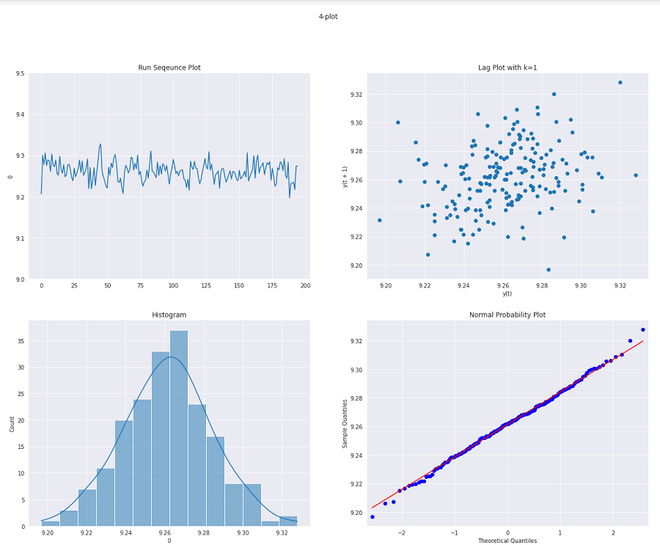Skip to content
Related Articles
4-Plot
• Last Updated : 09 Mar, 2021

A  4-plot is a collection of 4 different graphical Exploratory Data Analysis (EDA) tools, whose main motive is to test the assumptions that underlie most measurement processes.

The 4-plot consists of the following:

• Run plot: A run sequence plot is used to test fixed location and variations. It has the following axes:
• Vertical axis: Yi
• Horizontal axis: i
• Lag Plot: Lag plot is a type of scatter plot with one variable is lagged of the other. Here, lag means the value of the variable after some fixed amount of time. A log plot can be used to test the randomness of the process and can give important information about the distribution of the process.
• Vertical axis: Yi
• Horizontal axis: Yi-k
• Histogram: Histogram is the plot of values of data vs their frequency in the dataset. The histogram is used to know the distribution of the process i.e whether it is uniform, normal, etc.
• Vertical axis: counts/frequency/probability.
• Horizontal axis: Y
• Normal Probability: Normal probability plot is used to know how close the process distribution to normal distribution.
• Vertical axis: Ordered Yi
• Horizontal axis: The theoretical values from the normal distribution N(0,1).

4-plot can answer the following questions:

• Is the process in control, stable and predictable?
• Is the process drifting with respect to the location?
• Is the process drifting with respect to the variation?
• Are the data random?
• Is the observation related to an adjacent observation?
• If the distribution is not-random, then what is the distribution?
• Is sample mean a good estimator for the process, if not what is a good estimator?

Some assumption that can be verified with 4-plot are:

• Random generation.
• Fixed Distribution.
• The distribution having a fixed location
• The distribution having a fixed variation with time.

There are some underlying assumptions that follow the necessity for 4-plot:

• If the fixed location assumption holds, then the run sequence plot will be flat and non-drifting.
• If the fixed variation assumption holds then the vertical spread in the run sequence plot will be approximately the same over the entire horizontal axis.
• If the randomness assumptions hold then the lag plot will not form any type of structure.
• If the normal distribution assumption holds then the histogram will be bell-plot.

If all the above assumptions hold, then the process is in control.

### Implementation:

• In this implementation, we will also use statsmodels library as well as some common data science packages (Numpy, Pandas, and Seaborn). All these libraries are preinstalled in Colab and can be installed in the local environment with pip install.
• For this code, we will be using a heat flow meter dataset. The dataset can be downloaded from here.

## Python3

 `# code``import` `pandas as pd``import` `matplotlib.pyplot as plt``import` `seaborn as sns``import` `statsmodels.api as sm``%``matplotlib inline`` ` `heat_flow ``=` `pd.read_csv(``'heat_flow.txt'``, header ``=` `None``)``  ` `heat_flow.head()``sns.set_style(``'darkgrid'``)`` ` `# plot different components of 4 plot``fig, ax  ``=` `plt.subplots(``2``,``2``)``sns.lineplot(x ``=` `pd.Series(heat_flow.index),y ``=` `heat_flow[``0``],ax ``=` `ax[``0``,``0``])`` ` `ax[``0``,``0``].set_title(``'Run Seqeunce Plot'``)``pd.plotting.lag_plot(heat_flow[``0``],ax ``=` `ax[``0``,``1``])`` ` `ax[``0``,``1``].set_title(``'Lag Plot with k=1'``)``sns.histplot(heat_flow[``0``],kde ``=` `True``,ax ``=` `ax[``1``,``0``])`` ` `ax[``1``,``0``].set_title(``'Histogram'``)``sm.ProbPlot(heat_flow[``0``]).qqplot(line ``=``'s'``, ax ``=` `ax[``1``,``1``],color ``=` `'blue'``);`` ` `ax[``1``,``1``].set_title(``'Normal Probability Plot'``)``plt.show()`
```        0
0    9.206343
1    9.299992
2    9.277895
3    9.305795
4    9.275351```4-plot

• We can infer from the above 4-plot that:
• Here, the run sequence plot is quite flat and non-drifting. Hence, the fixed location assumption holds.
• The run sequence plot also has a quite similar vertical spread. Hence, the fixed variation assumption hold.
• Here, the lag plot does not generate any non-random pattern. Hence, we can assume that distribution is random.
• Here, the histogram generates quite symmetric bell-curve distribution. Hence, the process is normally distributed.
• Indeed, the above point can be confirmed with the normal probability plot generating scatter quite similar to normal distribution.

### References:My Personal Notes arrow_drop_up